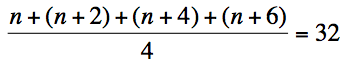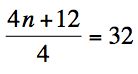# PSAT Math : Statistics

## Example Questions

### Example Question #1 : Arithmetic Mean

Ten students take an exam and score the following grades:

97

86

67

75

89

95

93

75

81

88

What is the mean score on the exam?

83.2

88

84.6

85

83.2

84.6

Explanation:

The mean, or average, score is determined by adding up all the scores and then dividing by the total number of tests:

(97+86+67+75+89+95+93+75+81+88) / 10 = 846 / 10 = 84.6

### Example Question #2 : Statistics

Find the mean in a given set of numbers:

1, 4, 8, 17, 8, 8, 15, 21, 32, 17

14.9

31

None of these

8

13.1

13.1

Explanation:

In order to find the mean, add all the numbers together (131) and divide by the number of items (10) = 13.1

### Example Question #11 : How To Find Arithmetic Mean

In a certain game, each of five players received a score between 0 and 100 inclusive. If their average (arithmetic mean) score was 50, what is the greatest possible number of the five players who could have received a score of 75?

Three

Five

None

Four

Two

Three

Explanation:

The question tells us that 50 was the average score of the 5 players, which means that 50 = total points / 5. So, the total points scored in the game must be 5 * 50 = 250.

Going through the answer choices, suppose all five players scored 75—then the total points would be 75 + 75 + 75 + 75 + 75 = 375, which is not 250, so five is not the correct answer.

Suppose four of the five players scored a 75—then the total points for players a, b, c, and d would be 75 + 75 + 75 + 75 = 300, and player e would have to score -50 for the total points to equal 250. Since player e's score cannot equal –50 (the problem says that scores are between 0 and 100), four is not the correct answer.

Now suppose that three of the four players scored a 75—then the total points for players a, b, and c would be 75 + 75 + 75 = 225, meaning d + e would have to equal 25 for the total points to equal 250. This scenario is possible in the game, if, for example, player d scored 12 points and player e scored 13 points. Therefore the greatest possible number of the 5 players who could have scored a 75 is three.

### Example Question #12 : Data Analysis

In a certain class, there are are 12 girls and 17 boys. If the average (arithmetic mean) height of the girls is g and the average (arithmetic mean) height of the boys is b, what is the average height of all of the girls and boys in the class?

(12g+17b)/29

(b+g)/29

29bg

(b+g)/2

b+g

(12g+17b)/29

Explanation:

Averages are calculated by the formula: TOTAL SUM FOR THE GROUP/ NUMBER OF ELEMENTS IN THE GROUP. We are told that the average for the 12 girls is g and the average for the 17 boys is b. Our formula for averages tells us that = TOTAL SUM OF GIRLS’ HEIGHTS / 12 and that = TOTAL SUM OF BOYS’ HEIGHTS / 17. Rearranging the terms, we see that the TOTAL SUM OF GIRLS’ HEIGHTS = 12g and the TOTAL SUM OF BOYS’ HEIGHTS =17b.

Again using our formula for averages, we see that the average height of all of the boys and girls in the class is given by the TOTAL HEIGHT FOR ALL BOYS AND GIRLS / TOTAL NUMBER OF BOYS AND GIRLS. We know the total height for all boys and girls will be the sum of the total girls' height and the total boys' height, or 12g +17b, and we know that the total number of boys and girls in the class is 12+17=29. Therefore, the average height for all boys and girls in the class is (12g +17b)/29.

### Example Question #13 : Data Analysis

If the average (arithmetic mean) of four consecutive odd integers is 32, what is the value of the least of the four integers?

31

29

27

21

33

29

Explanation:

Four consecutive odd integers can be expressed as n +(n +2)+(n +4)+(n +6), where n  is the least of the integers. If the mean of the 4 integers is 32, thenCollect like terms to findSimplifying gives n + 3 = 32 , meaning that n must be 29.

### Example Question #11 : Statistics

A class has test results of 98, 86, 72, 88, and 92. What score does Angie have to get on the test in order to make the average a 85?

60

74

87.2

63

90

74

Explanation:

With Angie's score there will be 6 total scores. 60*85(the average) gives a sum of scores as 510. Subtracting the scores of the other students gives a difference of 74. This means that Angie must score a 74 to have the class average be 85.

### Example Question #11 : Arithmetic Mean

If Mary traveled 116 miles on day 1, 130 miles on day 2, 114 miles on day 3. How many miles per day did she average?

118

115

116

114

120

120

Explanation:

To find an average you add all the values and divide by the number of values. 116+114+130 = 360. 360/3 = 120

### Example Question #13 : Statistics

The mean of 12, 14, 18, 20, and x is equal to 14. Solve for x.

20

6

14

5

12

6

Explanation:
1. Set up an equation to find the mean (or average) of the series of numbers.          (x + 64)/5 = 14     (64 = 12 + 14 + 18 + 20)
2. In order to solve for x, first multiply both sides by 5
3. x + 64 = 70
4. Subtract 64 from both sides.
5. x = 6.

### Example Question #12 : How To Find Arithmetic Mean

The St. Louis area has the following weather:

Monday            High Temperature  76     Low Temperature  51

Tuesday           High Temperature  82     Low Temperature  62

Wednesday     High Temperature  67     Low Temperature  37

What is the difference between the high temperature average and the low temperature average over the three days?

50

65

75

40

25

25

Explanation:

Average = sum of data points ÷ number of data points

High temperature average  = (76 + 82 + 67) ÷ 3 = 75

Low temperature average  = (51 + 62 + 37) ÷ 3 = 50

The difference between the two averages is 25.

### Example Question #14 : Statistics

In the following set of numbers, the arithmetic mean exceeds the mode by how much?

{2, 2, 4, 8, 10, 12, 20, 30}

4

8

9

6

2

9

Explanation:

The arithmetic mean is defined as:

The sum of a list of values divided by the number of values in the list.

Therefore for this problem, the arithmetic mean is:

(2+2+4+8+10+12+20+30) / 8 = (88/8) = 11

The mode is defined as the value that occurs the greatest number of times in a list of values.

In this case, it would be 2.

Therefore, the arithmetic mean (11) exceeds the mode (2) by 9.

### All PSAT Math Resources